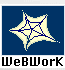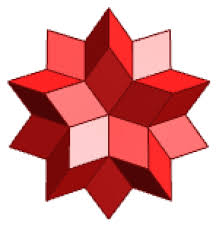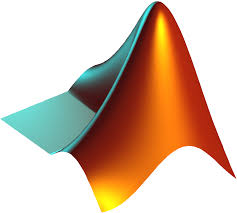## Resources

Below are links to various resources for the course.

### WeBWorKWeBWorK

#### Wolfram Alpha

Wolfram Alpha is an online answer engine that is very useful for mathematical problems. It can differentiate and integrate, plot functions and much more.Wolfram Alpha

#### Maple

Like Wolfram Alpha, Maple can be used for checking differentiation, plotting functions or visualizing surfaces in three-space. At Dartmouth there is licensed software available for students. If you would like to install Maple you can follow the instructions here:Maple

Below is a Quickstart Guide which lists various things you can do with Maple giving both the mathematical expression familiar to you as well as the syntactically correct command in Maple. To download the two worksheets (on integration and graphing) save the two files.

#### MATLAB

MATLAB, a contraction of "matrix laboratory", is a computing environment and programming language developed by MathWorks. It can be used in a similar fashion as Maple. Dartmouth has a site license for MATLAB. You can download it here:MATLAB